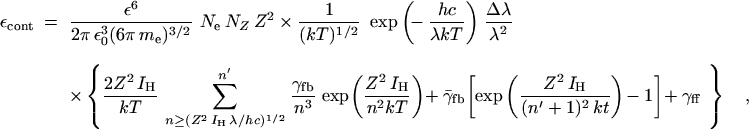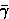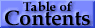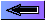• ### Hydrogenic Species

Precise quantum-mechanical calculations exist only for hydrogenic species. The total power εcont radiated (per unit source volume and per unit solid angle, and expressed in SI units) in the wavelength interval Δλ is the sum of radiation due to the recombination of a free electron with a bare ion (free-bound transitions) and bremsstrahlung (free-free transitions):(44)

where Ne is the electron density, NZ the number density of hydrogenic (bare) ions of nuclear charge Z, IH the ionization energy of hydrogen, n′ the principal quantum number of the lowest level for which adjacent levels are so close that they approach a continuum and summation over n may be replaced by an integral. (The choice of n′ is rather arbitrary; n′ as low as 6 is found in the literature.) γf b and γf f are the Gaunt factors, which are generally close to unity. (For the higher free-bound continua, starting with n′ + 1, an average Gaunt factorf b is used.) For neutral hydrogen, the recombination continuum forming H- becomes important, too .

In the equation above, the value of the constant factor is 6.065 × 10-55 W m4 J1/2 sr-1. [Numerical example: For atomic hydrogen (Z = 1), the quantity εcont has the value 2.9 W m-3 sr-1 under the following conditions: λ = 3 × 10-7 m; Δλ = 1 × 10-10 m; Ne (= NZ=1) = 1 × 1021 m-3; T = 12 000 K. The lower limit of the summation index n is 2; the upper limit n′ has been taken to be 10. All Gaunt factors γf b,f b, γf f have been assumed to be unity.]

• ### Many-Electron Systems

For many-electron systems, only approximate theoretical treatments exist, based on the quantum-defect method (for results of calculations for noble gases, see, e.g., Ref. ). Experimental work is centered on the noble gases . Modifications of the continuum by autoionization processes must also be considered.

Near the ionization limit, the f values for bound-bound transitions of a spectral series (n′ → ∞) make a smooth connection to the differential oscillator strength distribution df/dε in the continuum .